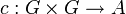# Alternating bihomomorphism of finitely generated abelian groups arises as skew of 2-cocycle

## Statement

### In terms of 2-cocycles

Suppose$G$ and$A$ are finitely generated abelian groups. Suppose$\lambda:G \times G \to A$ is an alternating bihomomorphism of groups from$G$ to$A$. Then, there exists a 2-cocycle for trivial group action$c:G \times G \to A$ such that$\operatorname{Skew}c = \lambda$, i.e.,:$\! c(g,h) - c(h,g) = \lambda(g,h) \ \forall \ g,h \in G$

### In terms of cohomology groups

Suppose$G$ and$A$ are finitely generated abelian groups. Consider the homomorphism:$H^2(G,A) \stackrel{\operatorname{Skew}}{\to} \bigwedge^2(G,A)$

which sends a cohomology class to the skew of any 2-cocycle representing it (that this is a homomorphism arises from the fact that skew of 2-cocycle for trivial group action of abelian group is alternating bihomomorphism). Then, this homomorphism is surjective, i.e., every alternating bihomomorphism arises from some cohomology class.

## Facts used

1. Structure theorem for finitely generated abelian groups
2. Symplectic decomposition of an alternating bilinear form taking values in a local principal ideal ring
3. Orthogonal direct sum of cocycles is cocycle
4. Symplectic decomposition of an alternating bilinear form taking values in integers

## Proof

### First part:reduction to the case where$A$ is either infinite cyclic or cyclic of prime power order

We first show that the problem can be reduced to the case that$A$ is a cyclic group.

Given: Finitely generated abelian groups$A$ and$G$, an alternating bihomomorphism$\lambda:G \times G \to A$.

To prove: Assuming that we can solve the problem if$A$ were replaced by an infinite cyclic group or a cyclic group of prime power order, we can solve the problem in the general case, i.e., we can find a 2-cocycle$c$ such that$\operatorname{Skew}(c) = \lambda$.

Proof:

Step no. Assertion/construction Facts used Given data/assumptions used Previous steps used Explanation
CR 1 We can find cyclic groups$A_1,A_2, \dots, A_n$ such that$A = A_1 \oplus A_2 \oplus \dots \oplus A_n$, such that each$A_i$ is either infinite cyclic or cyclic of prime power order Fact (1)$A$ is finitely generated and abelian Given+Fact direct
CR 2 Let$\pi_i$ be the projection of$A$ on to the factor$A_i$. Then, define$\lambda_i = \pi_i \circ \lambda$. Each$\lambda_i$ is an alternating bihomomorphism from$G$ to$A_i$. Step (CR1)
CR3 We can find cocycles$c_i:G \times G \to A_i$such that$\operatorname{Skew}(c_i) = \lambda_i$. Assumption that we can solve the reduced problem. Step (CR1): Each$A_i$ is cyclic; Step (CR2):$\lambda_i$ is an alternating bihomomorphism
CR4 Consider the mapping$c = c_1 \oplus c_2 \oplus \dots \oplus c_n$.$c$ is a 2-cocycle$G \times G \to A$ and$\operatorname{Skew}(c) = \lambda$. Step (CR3)